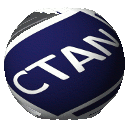# yquant

Typesetting quantum circuits in a human-readable language

yquant is a package that allows to quickly draw quantum circuits. It bridges the gap between the two groups of packages that already exist: those that use a logic-oriented custom language, which is then translated into by means of an external program; and the pure versions that mainly provide some macros to allow for an easier input. yquant is a pure- solution - i.e., it requires no external program - that introduces a logic oriented language and thus brings the best of both worlds together. It builds on and interacts with TiKZ, which brings an enourmous flexibility for customization of individual circuit.

Important features in the latest updates (for a much more complete list, see the documentation):

• Support for vertical circuit layout (since 0.7)
• Support for a simplified drawing pipeline (since 0.7)
• Support the beamer package (since 0.6)
• New text gate, which replaces the common [draw=none] box situation (since 0.6)
• Integration of all qpic examples in the manual, showcasing some very advanced circuits (since 0.6)
• Simple interface for circuit equations, no more subcircuit hassling (since 0.5)
• Measurement outputs can now directly control other gates, i.e., with a vertical classical line emanating from the measurement (since 0.4)
• Automatically adjust vertical positions of wires also for multi-register gates (since 0.4-alpha)
• Vertical alignment actually works for subcircuits (since 0.4-alpha)
• Directly support circuits written in the qasm language (since 0.3)
• Simple declaration of custom gates (since 0.2.1)
• Support for subcircuits, though vertical alignment may be messed up (since 0.2)
• Load circuits from files (since 0.2)
• Native support for non-contiguous multi-qubit gates (since 0.1.2)
• Extensive user-friendly support for register name ranges, lists (since 0.1.1)

A detailed reference with lots of examples is provided in the PDF version of this Readme. We will sketch some basic usage.

Support the development:

## Examples

### Simple teleportation circuit\begin{tikzpicture}
\begin{yquant}
qubit {$\ket{\reg_{\idx}}$} q;

h q;
cnot q | q;
cnot q | q;
h q;
measure q[0-1];

z q | q;
x q | q;
\end{yquant}
\end{tikzpicture}


### Three-qubit phase estimation circuit with QFT and controlled-U\begin{tikzpicture}
\begin{yquant}
qubit {$\ket{j_{\idx}} = \ket0$} j;
qubit {$\ket{s_{\idx}}$} s;

h j;
box {$U^4$} (s) | j;
box {$U^2$} (s) | j;
box {$U$} (s) | j;
h j;
box {$S$} j | j;
h j;
box {$T$} j | j;
box {$S$} j | j;
h j;
measure j;
\end{yquant}
\end{tikzpicture}


### Three-qubit FT QEC circuit with syndrome measurement\begin{tikzpicture}
\begin{yquant}
qubit {$\ket{q_{\idx}}$} q;
qubit {$\ket{s_{\idx}} = \ket0$} s;
cbit {$c_{\idx} = 0$} c;

h s;
cnot s | s;
cnot s | q;
cnot s | q;
cnot s | s;
h s;
measure s;
cnot c | s;
cnot c | s;

init {$\ket0$} s;
h s;
cnot s | s;
cnot s | q;
cnot s | q;
cnot s | s;
h s;
measure s;

box {Process\\Syndrome} (s, c);
box {$\mathcal R$} (q) | s, c;
\end{yquant}
\end{tikzpicture}


### Error correction% \usetikzlibrary{quotes}
\begin{tikzpicture}
\begin{yquant}
qubit {} msg;
nobit syndrome;

[this subcircuit box style={dashed, "Syndrome Measurement"}]
subcircuit {
qubit {} msg;
[out]
qubit {$\ket0$} syndrome;

cnot syndrome | msg;
cnot syndrome | msg;
cnot syndrome | msg;
cnot syndrome | msg;
cnot syndrome | msg;
cnot syndrome | msg;

dmeter {$M_{\symbol{\numexpra+\idx}}$} syndrome;
} (msg[-2], syndrome[-2]);

["Recovery"]
box {$\mathcal R$} (msg) | syndrome;
\end{yquant}
\end{tikzpicture}


### Lots of controls\begin{tikzpicture}
\begin{yquant*}
zz (a[0, 2]);
cnot a ~ a;
zz (a[2, 3]);
h a | a ~ a;
measure a[2, 3];
box {$U$} (a[0, 1]) | a ~ a;
\end{yquant*}
\end{tikzpicture}


### Circuit equations% \useyquantlanguage{groups}
\begin{tikzpicture}
\begin{yquantgroup}
\registers{
qubit {} q;
}
\circuit{
h -;
cnot q | q;
h -;
}
\equals
\circuit{
cnot q | q;
}
\end{yquantgroup}
\end{tikzpicture}
`